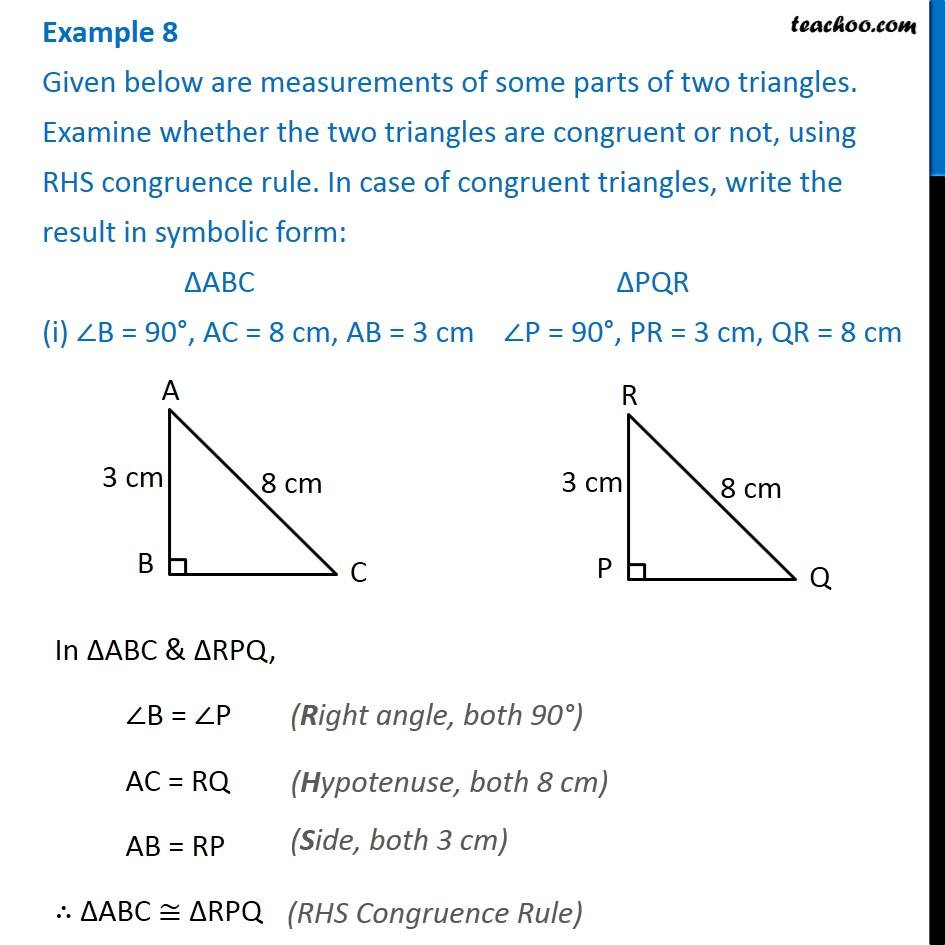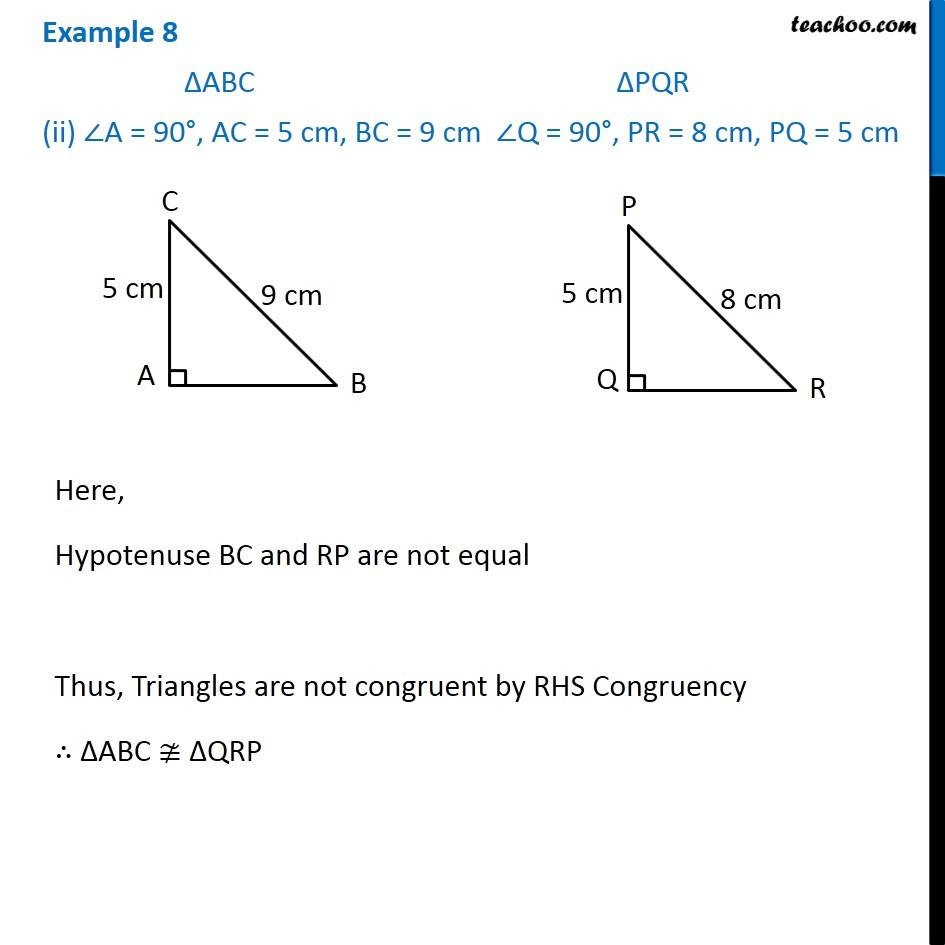1. Chapter 7 Class 7 Congruence of Triangles
2. Concept wise
3. RHS Congruency Criteria

Transcript

Example 8 Given below are measurements of some parts of two triangles. Examine whether the two triangles are congruent or not, using RHS congruence rule. In case of congruent triangles, write the result in symbolic form: ∆ABC ∆PQR (i) ∠B = 90°, AC = 8 cm, AB = 3 cm ∠P = 90°, PR = 3 cm, QR = 8 cmIn ∆ABC & ∆RPQ, ∠B = ∠P AC = RQ AB = RP ∴ ∆ABC ≅ ∆RPQ Example 8 ∆ABC ∆PQR (ii) ∠A = 90°, AC = 5 cm, BC = 9 cm ∠Q = 90°, PR = 8 cm, PQ = 5 cm Here, Hypotenuse BC and RP are not equal Thus, Triangles are not congruent by RHS Congruency ∴ ∆ABC ≇ ∆QRP

RHS Congruency Criteria